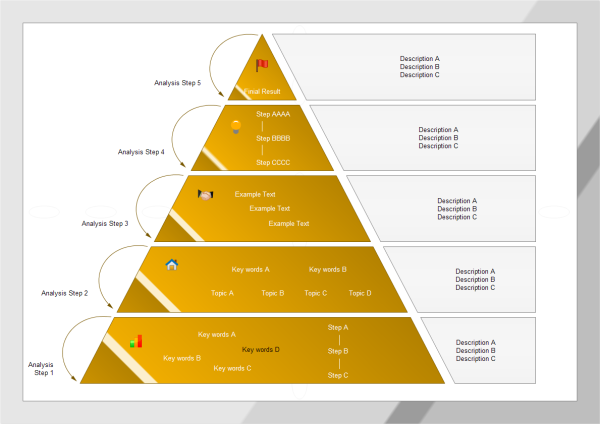# Triangle Diagram Templates and Examples

> Triangle Diagram Templates and Examples
Posted by James Freeman |
Here shows a triangle diagram sample drawn with Edraw triangle diagram software. Using this easily customizable triangle diagram template you can represent any existing triangle diagram.

### Triangle Diagram Templates

A triangle diagram template is ready to use for a simple timeline template. Use this triangular scheme template to create your own marketing triangle chart for illustration of presentation template triangle graph.

The triangle diagram template can reduce the amount of time to create great triangle diagram by using built in symbols right next to the canvas. You can download and modify this template for your own use.